# Count Number of Nodes in a Binary Tree

In this article, we will solve the most asked coding interview problem: Count Number of Nodes in a Binary Tree

Write a program that calculates the number of nodes in a complete binary tree.

```Example: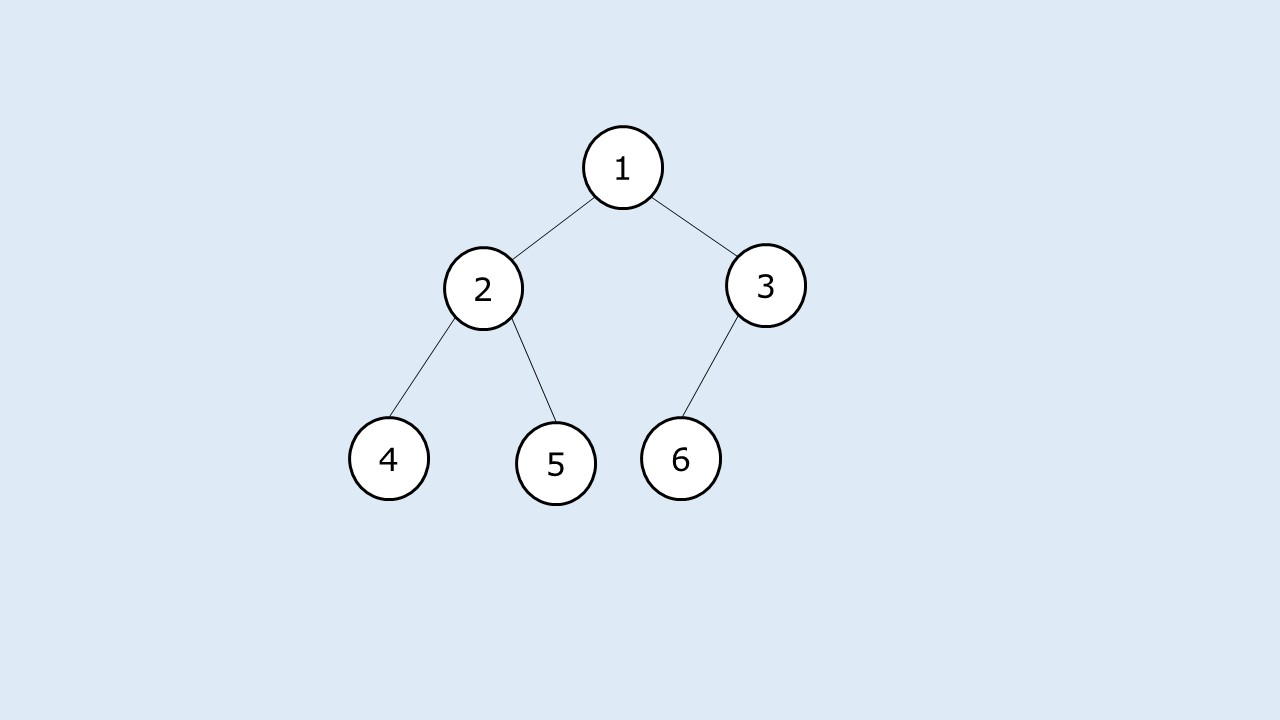Output:

The total number of nodes in the given complete binary tree are: 6
```

What is a complete binary tree?

A complete binary tree is a binary tree whose:

• All levels except the last one are completely filled. The last level may or may not be completely filled.
• Nodes in the last level are as left as possible.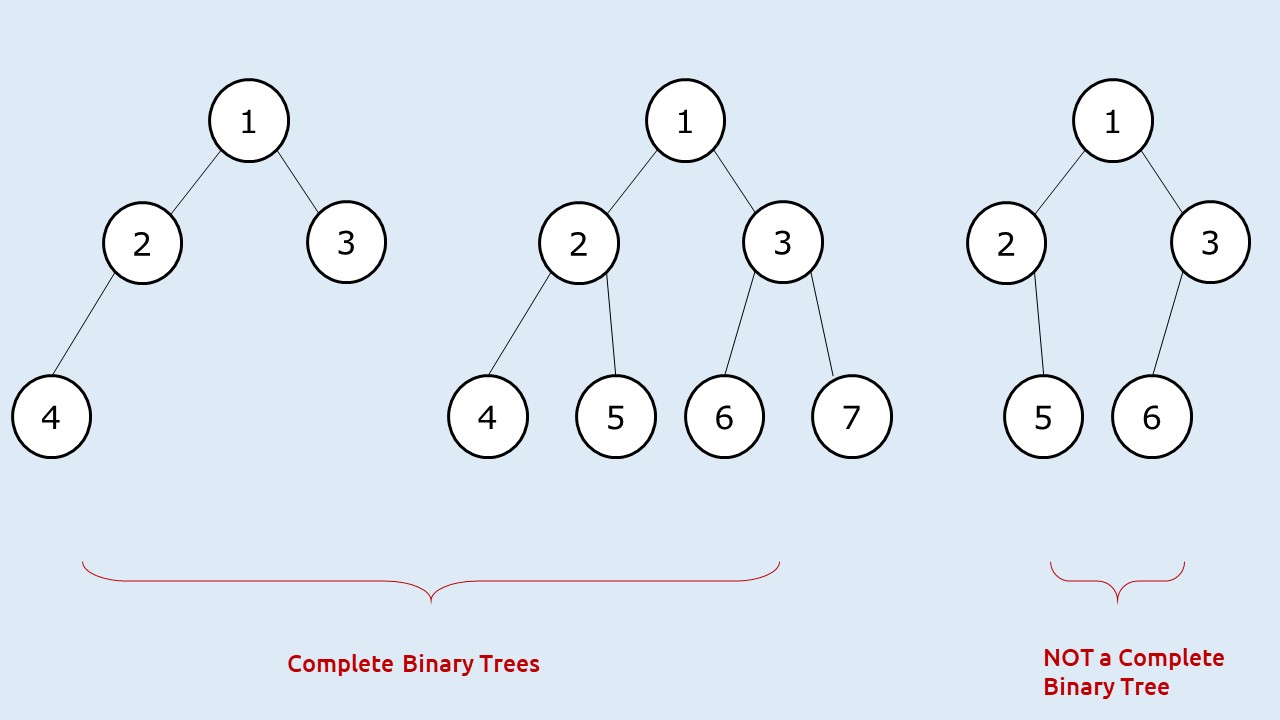Disclaimer: Don’t jump directly to the solution, try it out yourself first.

### Solution :

Solution 1: Using any tree traversal – O(N) Approach

Approach:

The first approach that comes to our mind is to use any tree traversal ( say inorder), and count the number of nodes as we are traversing the tree.

Code:

## C++ Code

``````#include <bits/stdc++.h>

using namespace std;

struct node {
int data;
struct node * left, * right;
};

struct node * newNode(int data) {
struct node * node = (struct node * ) malloc(sizeof(struct node));
node -> data = data;
node -> left = NULL;
node -> right = NULL;

return (node);
}

void inOrderTrav(node * curr, int& count) {
if (curr == NULL)
return;

count++;
inOrderTrav(curr -> left, count);
inOrderTrav(curr -> right, count);
}

int main() {

struct node * root = newNode(1);
root -> left = newNode(2);
root -> right = newNode(3);
root -> left -> left = newNode(4);
root -> left -> right = newNode(5);
root -> right -> left = newNode(6);

int count = 0;
inOrderTrav(root, count);

cout << "The total number of nodes in the given complete binary tree are: "
<<count;
return 0;
}
``````

Output:

The total number of nodes in the given complete binary tree are: 6

Time Complexity: O(N).

Reason: We are traversing for every node of the tree

Space Complexity: O(logN)

Reason: Space is needed for the recursion stack. As it is a complete tree, the height of that stack will always be logN.

## Java Code

``````import java.util.*;

class Node {
int data;
Node  left, right;
Node(int data)
{
this.data=data;
left=null;
right=null;
}
}

class TUF{
static void inOrderTrav(Node  curr, int count[]) {
if (curr == null)
return;

count++;
inOrderTrav(curr . left, count);
inOrderTrav(curr . right, count);
}

public static void main(String args[]) {

Node  root = new Node(1);
root . left = new Node(2);
root . right = new Node(3);
root . left . left = new Node(4);
root . left . right = new Node(5);
root . right . left = new Node(6);

int count[]=new int;
inOrderTrav(root, count);

System.out.println("The total number of nodes in the given complete binary tree
are: "+count);
}
}
``````

Output:

The total number of nodes in the given complete binary tree are: 6

Time Complexity: O(N).

Reason: We are traversing for every node of the tree

Space Complexity: O(logN)

Reason: Space is needed for the recursion stack. As it is a complete tree, the height of that stack will always be logN.

Approach 2: Efficient Approach – O(log^2 N) solution

We will now try to form an algorithm that takes less than O(N) time.

We are given a complete binary tree that has two special properties:

• All levels except the last one are completely filled
• Last level nodes are as left as possible.

If we get to know the height of the binary tree (say h), we can find out the maximum number of nodes that can be present by the formula: 2h – 1. So there is some relation between height and number of nodes.

How to find the height in less than O(N) time? As the given tree is a complete binary tree, we can find the height of the binary tree by just finding the left height of the tree, as the left height will always be equal to the height of the tree.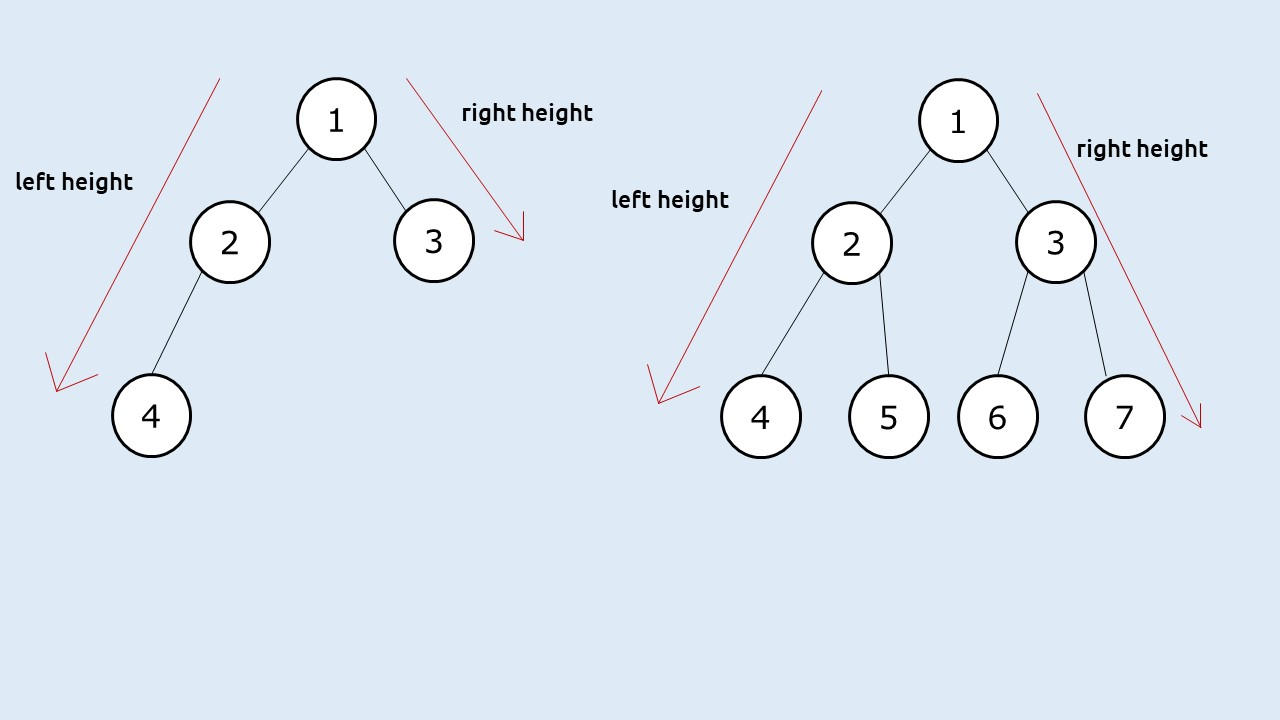If the last level of the binary tree is completely filled( a perfect binary tree), then this left height will give us the total count of nodes present by the formula: 2h – 1. Now how to check whether the last level is completely filled? A simple way is to find the right height of the given tree, then:

• If leftHeight == rightHeight, then the last level nodes are completely filled
• If leftHeight != rightHeight, then the last level nodes are not completely filled

In the second case, when leftHeight != rightHeight, we can take the help of recursion and say to recursively find the number of nodes in the left subtree (say leftNodes) and in the right subtree(say rightNodes) and then return 1 + leftNodes + rightNodes.

Approach:

The algorithm steps can be stated as :

• Set a recursive function to calculate the number of nodes.
• In the recursive function, calculate leftHeight and the right Height of the tree from the given node.
• If leftHeight == rightHeight, return 2leftHeight – 1.
• If leftHeight != rightHeight, recursively call the function to calculate nodes in left subtree(leftNodes) and the right subtree(rightNodes) and return 1+leftNodes+rightNodes.

Dry Run:

Let us take a bigger example: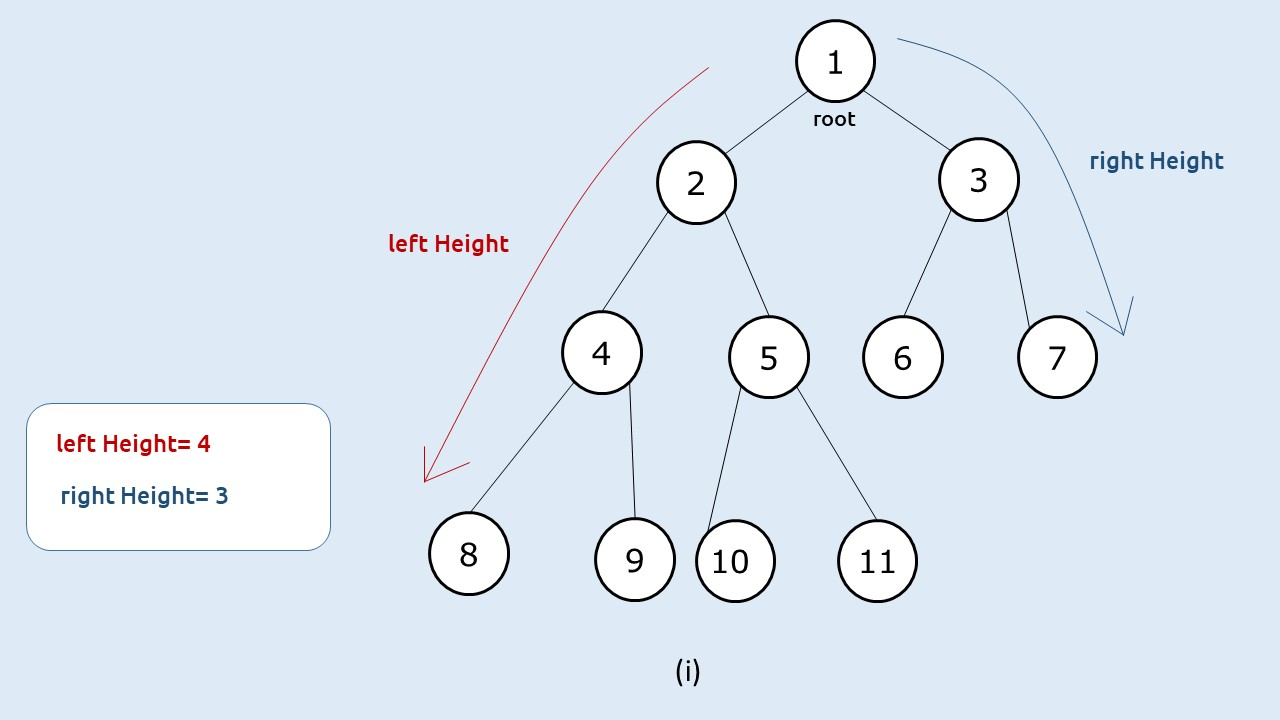(i) First when we are at the root node, we will find the left height and the right height. In this case, as leftHeight != rightHeight, we will recursively call the function to find leftNodes and rightNodes.(ii) Then, we find the answer for the first recursive call of step 1. When root = 2, we see that the left height == right Height(both equal to 3), so we simply return 2^(3) – 1 (which is 7).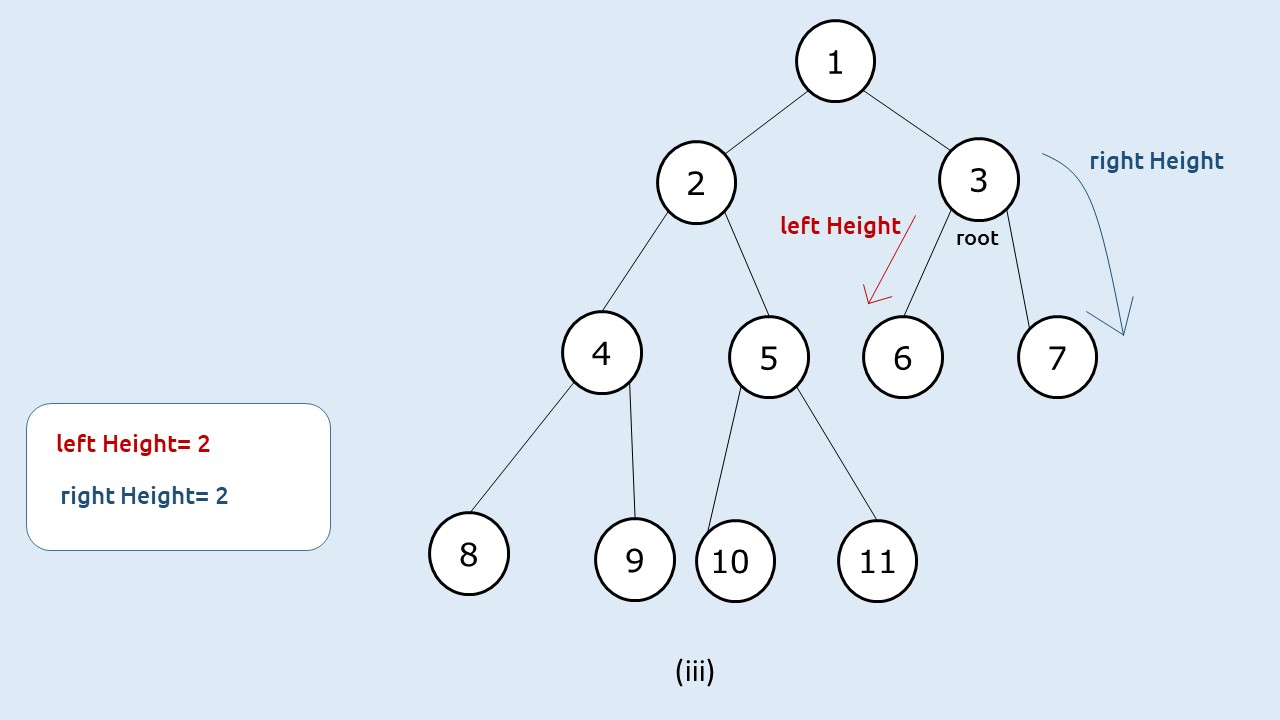(iii) Then, we find the answer for the second recursive call of step 1. When root = 3, we see that the left height == right Height(both equal to 2), so we simply return 2^(2) – 1 (which is 3).

(iv) At last, we will return to the root node of the tree (node 1) and we will return 1+ leftNodes + rightNodes, i.e 1+7+3 = 11.

Code:

## C++ Code

``````#include <bits/stdc++.h>

using namespace std;

struct node {
int data;
struct node * left, * right;
};

struct node * newNode(int data) {
struct node * node = (struct node * ) malloc(sizeof(struct node));
node -> data = data;
node -> left = NULL;
node -> right = NULL;

return (node);
}

void inOrderTrav(node * curr, int& count) {
if (curr == NULL)
return;

count++;
inOrderTrav(curr -> left, count);
inOrderTrav(curr -> right, count);
}

int findHeightLeft(node* cur) {
int hght = 0;
while(cur) {
hght++;
cur = cur->left;
}
return hght;
}
int findHeightRight(node* cur) {
int hght = 0;
while(cur) {
hght++;
cur = cur->right;
}
return hght;
}
int countNodes(node* root) {
if(root == NULL) return 0;

int lh = findHeightLeft(root);
int rh = findHeightRight(root);

if(lh == rh) return (1<<lh) - 1;

int leftNodes = countNodes(root->left);
int rightNodes = countNodes(root->right);

return 1 + leftNodes + rightNodes;
}

int main() {

struct node * root = newNode(1);
root -> left = newNode(2);
root -> right = newNode(3);
root -> left -> left = newNode(4);
root -> left -> right = newNode(5);
root -> right -> left = newNode(6);
root -> right -> right = newNode(7);
root -> left -> left -> left = newNode(8);
root -> left -> left -> right = newNode(9);
root -> left -> right -> left = newNode(10);
root -> left -> right -> right = newNode(11);

cout << "The total number of nodes in the given complete binary tree are: "
<<countNodes(root);
return 0;
}
``````

Output:

The total number of nodes in the given complete binary tree are: 11

Time Complexity: O(log^2 N).

Reason: To find the leftHeight and right Height we need only logN time and in the worst case we will encounter the second case(leftHeight!=rightHeight) for at max logN times, so total time complexity will be O(log N * logN)

Space Complexity: O(logN)

Reason: Space is needed for the recursion stack when calculating height. As it is a complete tree, the height will always be logN.

## Java Code

``````import java.util.*;

class Node {
int data;
Node  left, right;
Node(int data)
{
this.data=data;
left=null;
right=null;
}
}

class TUF{
static int findHeightLeft(Node cur) {
int hght = 0;
while(cur!=null) {
hght++;
cur = cur.left;
}
return hght;
}

static int findHeightRight(Node cur) {
int hght = 0;
while(cur!=null) {
hght++;
cur = cur.right;
}
return hght;
}

static int countNodes(Node root) {
if(root == null) return 0;

int lh = findHeightLeft(root);
int rh = findHeightRight(root);

if(lh == rh) return (1<<lh) - 1;

int leftNodes = countNodes(root.left);
int rightNodes = countNodes(root.right);

return 1 + leftNodes + rightNodes;
}

public static void main(String args[]) {

Node root = new Node(1);
root . left = new Node(2);
root . right = new Node(3);
root . left . left = new Node(4);
root . left . right = new Node(5);
root . right . left = new Node(6);
root . right . right = new Node(7);
root . left . left . left = new Node(8);
root . left . left . right = new Node(9);
root . left . right . left = new Node(10);
root . left . right . right = new Node(11);

System.out.println("The total number of nodes in the given complete binary tree
are: "+countNodes(root));
}
}
``````

Output:

The total number of nodes in the given complete binary tree are: 11

Time Complexity: O(log^2 N).

Reason: To find the leftHeight and right Height we need only logN time and in the worst case we will encounter the second case(leftHeight!=rightHeight) for at max logN times, so total time complexity will be O(log N * logN)

Space Complexity: O(logN)

Reason: Space is needed for the recursion stack when calculating height. As it is a complete tree, the height will always be logN.

Special thanks to Anshuman Sharma for contributing to this article on takeUforward. If you also wish to share your knowledge with the takeUforward fam, please check out this article